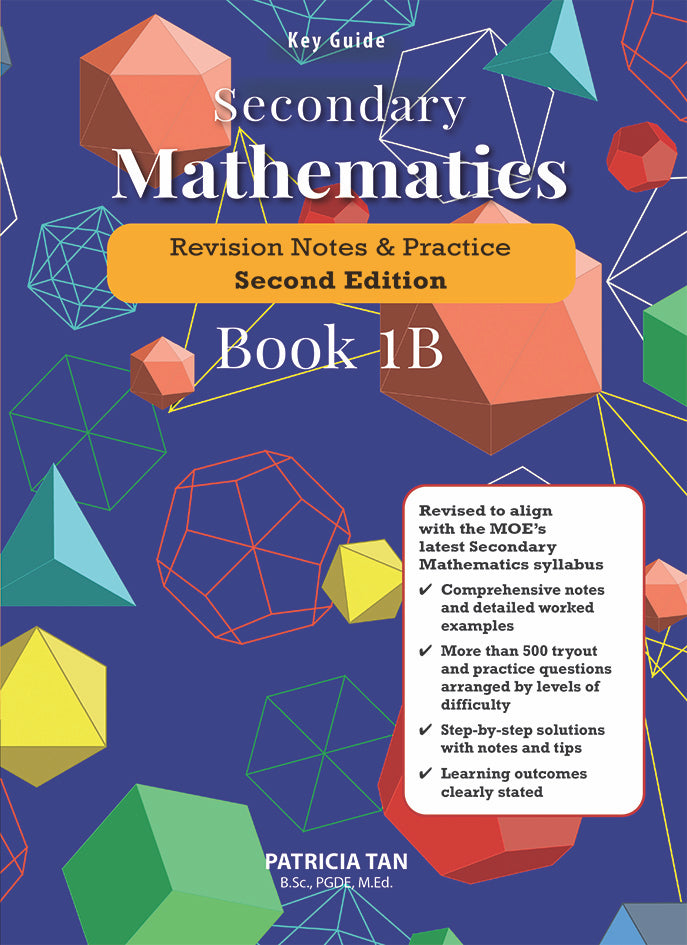1/ 4

# Key Guide Secondary Mathematics Revision Notes & Practice Book 1B (Second Edition)

\$1013
\$000
Quantity
Only 2 left
Secure payments

Key Guide: Secondary Mathematics Revision & Practicesis a two-volume guide (Books 1Aand 1B) designed specifically for the student who self-studies. ThisSecond Editionis revised to align with the latest Secondary Mathematics syllabus issued by the Ministry of Education, Singapore. A comprehensive set of notes, worked examples and practice questions with complete solutions are presented to help the student better understand the fundamentals in mathematics, learn to manipulate mathematical objects as well as revise in a more effective and efficient manner.

Unique features in each chapter of the book include:

1. Learning Outcomes:These help the student focus on the key learning concepts of each topic.
2. Notes:They explain concepts and consolidate learning.
3. Worked Examples:They illustrate the mathematical concepts presented and help the student see how mathematical concepts are applied.
4. Try it Out!:The questions in this section are designed to dipstick and reinforce learning immediately after a concept, sub-topic or skill.
5. Tips and Notes:These useful bubbles highlight manipulations, strategies and common pitfalls that students may make in the course of their learning.
6. Practice Time:Questions are categorised into three levels of learning Basic, Intermediate and Advance to help the student work through, develop and strengthen his or her understanding of mathematical concepts.
7. Step-by-step Solutions with Tips and Notes:Detailed solutions guide the self-study student to work out the most efficient solution to the practice questions.